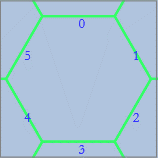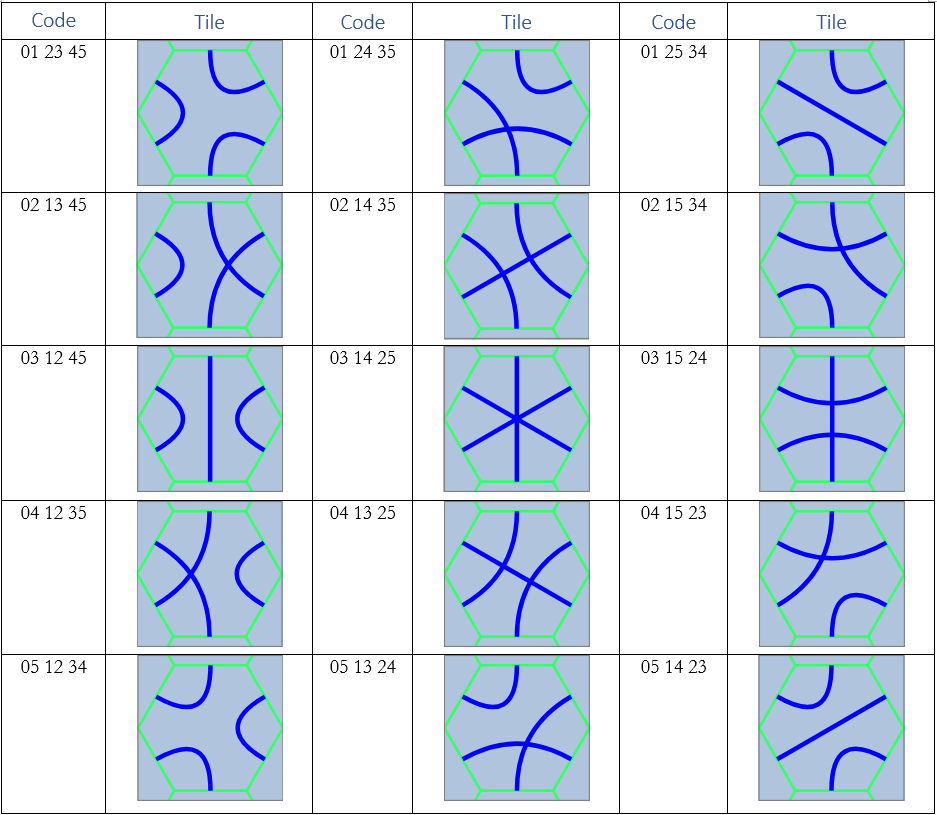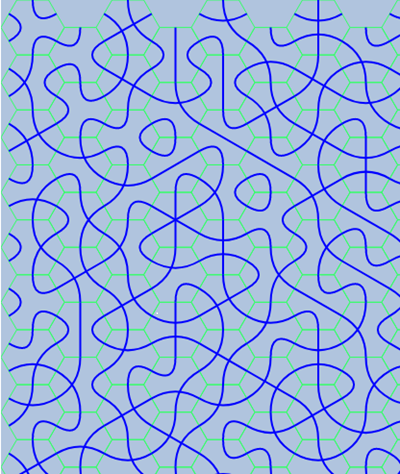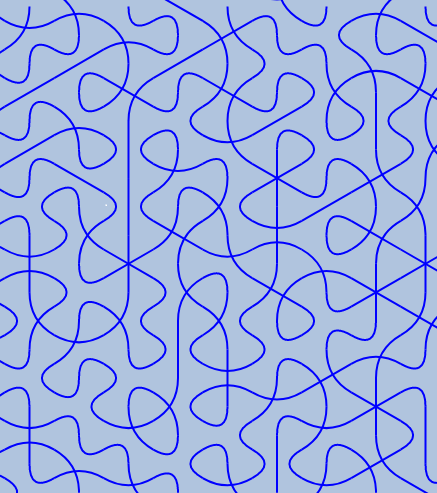(updated )

new cipher font (Steganography) Hex-Truchet

Truchet tiles are square tiles decorated with patterns. When placed in a square tiling of the plane, they can form varied patterns.
Original Truchet tiles are split along the diagonal into two triangles of contrasting colors. The tile has four possible orientations.
A second common form of the Truchet tiles decorates each tile with two quarter-circles connecting the midpoints of adjacent sides. Each such tile has two possible orientations.
Exist tiles in the form of a white square with a black diagonal. As with the quarter-circle tiles, each such tile has two orientations.
See Truchet tiles with images at wikipedia.

If we take a hexagonal tiling instead of a square one, each tile will have 6 edges. Thus, a Truchet hexagonal tile consists of 3 arcs/lines connecting a pair of edges. Let's take hexagonal tiles with a flat top. The numbering order of the edges is clockwise from the top edge.We have 5 options for choosing the first pair of 6 edges. And then 3 options for choosing the second pair of the 4 remaining edges. The last two edges make up the third pair without any options. Thus, there are a total of 15 hexagonal tiles.
The table below shows all the 15 tiles, with codes (3 pairs of edges).Put random tiles on the grid and we get different sets of curves:Repeated in consistent order they may form some patterns:How it looks without the underlying hex grid:The wikipedia article says: “… and the orientation of each tile can be used to visualize information associated with the tile's position within the tiling”. So we have 15 items, we can use them to encode the English alphabet, some punctuation and numbers.

See the next part: new cipher font (Steganography) Hex-Truchet - part 2, encoding

Truchet tiles - Wikipedia
EN.WIKIPEDIA.ORG
Moera
WEB.MOERA.ORG
💡👍2